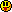ForumCS2DScriptsMonster size affects nothing in RPG TibiaMonster size affects nothing in RPG Tibia

6 replies
Goto Page1Goo
User
OfflineHi, I'm working on an RPG script because I'm not good enough at Lua to create my ownI have different monsters with different image sizes but when I try to change their
self.size
it doesn't affect anything.
I've searched throughout the whole script and it's only used here:
Code:
1
2
3
4
5
6
7
8
9
10
--[[
function occupied(x,y,monsterid)
for n, m in pairs (MONSTERS) do
if monsterid ~= n and math.sqrt(math.pow(math.abs(m.x-x),2) + math.pow(math.abs(m.y-y),2)) <= m.size then
return true
end
end
return false
end
]]

That function isn't even used.

I suppose
self.size
is supposed to be checked in this hook:
Code:
1
2
3
4
5
6
7
8
9
10
11
12
13
14
15
16
17
18
19
20
21
22
23
24
25
26
function RPG.attackHookMonster(id)
if gettile(PLAYERS[id].x, PLAYERS[id].y).SAFE or gettile(PLAYERS[id].x, PLAYERS[id].y).NOMONSTERS then
return
end
if inarray({400, 401, 402, 403, 404}, PLAYERS[id].Equipment) then
hudMsg(id,"You may not attack on a horse.","note")
return
end
local weapon, closest = player(id, 'weapontype')
for _, m in ipairs(MONSTERS) do
local x, y = player(id, 'x'), player(id, 'y')
local dist = math.sqrt((m.x-x)^2+(m.y-y)^2)
if dist <= (closest and closest or (CONFIG.WEAPONRANGE[weapon] or CONFIG.WEAPONRANGE)) then
local rot = player(id, 'rot')
if math.abs(math.rad(rot) - math.atan2(y-m.y, x-m.x) + math.pi/2)%(2*math.pi) <= (CONFIG.WEAPONWIDTH[weapon] or CONFIG.WEAPONRANGE) then
if free_line(player(id,"x"),player(id,"y"),m.x,m.y) then
closest = {m, dist}
end
end
end
end
if closest then
closest:damage(id,math.floor(((PLAYERS[id].tmp.atk*10)/closest.def)), weapon)
end
end

But I don't know where.
I've tried adding it to the function in random places but either it's not the right way or I've done it wrong.

Another place where it could be placed in is this function where I used to use the occupied function until it caused some issues and I was told I better off not use it:
Code:
1
2
3
4
5
6
7
8
9
10
11
12
13
14
15
16
17
18
19
20
21
22
23
24
25
function Monster:pos(x, y)
if not x and not y then
return self.x, self.y
else
--if not occupied(x,y,self.id) then
self.x, self.y = x or self.x, y or self.y
--end
for all = 1, 32 do
if player(all,"exists") and getHealth(all) > 0 then
if player(all,"x") >= self.x-800 and player(all,"x") <= self.x+800 and player(all,"y") >= self.y-800 and player(all,"y") <= self.y+800 then
imagepos(self.image, self.x, self.y, self.imgang)
if not self.alpha then
self.alpha=1
end
imagealpha(self.image, self.alpha)
break
end
end
if all == 32 then
imagealpha(self.image, 0)
end
end
end
return true
end

Does anyone have a clue on what I'm supposed to do to fix it?
Yates
Reviewer
OfflineThe existing code will not work if you simply decide to add it to the monster position function. You'll end up with monsters spawning on top of eachother that are unable to move because their path is obstructed. You also will have to change the hit detection, which wouldn't be all too hard, but I would recommend changing it completely by using the image hitzones instead.

I could probably find my old monsters file if you'd like, but I won't be cutting out bits to suit your needs, I'll just post it all and you can decide what you want to use.

Let me know if you're interested and I'll go searching tonight on one of my drives.
Goo
User
OfflineWell since the first paragraph of words you said left me in confusion I'd like to see what you're talking about on the second one!

But if you'll give me the whole file how will I know which part affects the size? Should I just use all of it or should I try replacing functions one by one hoping it'll work?

Maybe searching for
.size
inside your file and moving the appropriate functions to replace with mine?
Yates
Reviewer
OfflineI'll have to check for you tomorrow, will give you a general explanation of where I changed what if I can recall. I'll set an alarm to remind me to check, will be around this time, no worries.
Goo
User
OfflineOK I'll waitEDIT: I need the
self.size
to work so I can have higher hitboxes on monsters but if you know how to have a hitbox based on the monster's image size it would probably be better and easier because I won't have to go through each monster setting up it's own
self.size
.
edited 2×, last 26.09.18 04:38:20 am
Yates
Reviewer
OfflineMy apologies, I cannot find the version where I remade the monster functions, I can only find one from 2015 where I only checked the occupied space:

Code:
1
2
3
4
5
6
7
8
9
10
11
12
13
14
15
16
17
18
19
20
21
22
23
24
25
26
27
28
29
30
31
function Monster:pos(x, y)
if not x and not y then
return self.x, self.y
else
if not occupied(x,y,self.id) then
self.x, self.y = x or self.x, y or self.y
end
for all = 1, 17 do
if player(all,"exists") and player(all,"health") > 0 then
if player(all,"x") >= self.x-800 and player(all,"x") <= self.x+800 and player(all,"y") >= self.y-800 and player(all,"y") <= self.y+800 then
imagepos(self.image, self.x, self.y, self.imgang)
imagealpha(self.image, 1)
break
end
end
if all == 17 then
imagealpha(self.image, 0)
end
end
end
return true
end

function occupied(x,y,monsterid)
for n, m in pairs (MONSTERS) do
if monsterid ~= n and math.sqrt(math.pow(math.abs(m.x-x),2) + math.pow(math.abs(m.y-y),2)) <= m.size then
return true
end
end
return false
end

Within the position function it checks whether the space is occupied or not. It also contains a neat for loop that's so super-duper-awesome and why did I even.. In any case, it checks whether a player is nearby, if no one is, the image position will not change. This helped a lot of lag players were experiencing and also stops spectators taking a look around your map to see what monsters lurk around.

So this won't really help your issue with the monster sizes, but at least you won't have monsters walking over one another any more (yay!)Goo
User
OfflineThe only differences between my function and yours is that yours doesn't include this:

Code:
1
2
3
if not self.alpha then
self.alpha=1
end

below line 10.

And the fact you're using the occupied function while I'm not.
I'll give the occupied function another go and see what happens.

I now remember why I removed it, I have bosses in the script that are supposed to summon "minions".
The minions are spawned on top of the bosses which prevent the bosses from being able to move, the only way I can probably solve this is if I make bosses ignore the occupied function, not sure if that's a good idea though...

Well I've made bosses and minions ignore that function so now only monsters care about it, makes boss fights a little easier.

I've changed my occupied function to this:
Code:
1
2
3
4
5
6
7
8
function occupied(x,y,monsterid)
for n, m in pairs (MONSTERS) do
if monsterid ~= n and (math.sqrt(math.pow(math.abs(m.x-x),2)) <= m.width and math.sqrt(math.pow(math.abs(m.y-y),2)) <= m.height) then
return true
end
end
return false
end

Is there a way to add
m.width
and
m.height
to the attack hook so they'll have a bigger hitbox?
edited 1×, last 27.09.18 05:52:10 am1﻿